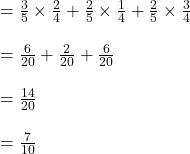## a bag contain 3 black balls and 2 white balls. 1. A ball is taken from the black and then replaced, a second is taken. what is the pro

Question

a bag contain 3 black balls and 2 white balls.
1. A ball is taken from the black and then replaced, a second is taken. what is the probabilities that.

(a) there are both black,
(b)one is black one is white,
(c) at lease one is black,
(d) at most one is one is black.

2. find out if all the balls are chosen without replacement.

please kindly solve with explanation. thank you.​

in progress 0
6 months 2021-07-25T21:19:44+00:00 1 Answers 5 views 0

## Answers ( )

Step-by-step explanation:

Total number of balls = 3 + 2 = 5

1)

a)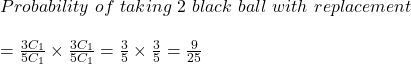b)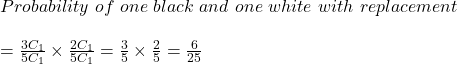c)

Probability of at least one black( means BB or BW or WB)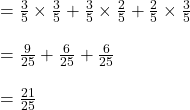d)

Probability of at most one black ( means WW or WB or BW)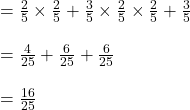2)

a) Probability both black without replacement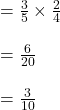b) Probability  of one black and one whitec) Probability of at least one black ( BB or BW or WB)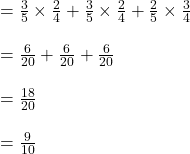d) Probability of at most one black ( BW or WW or WB)